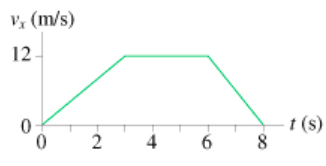# Problem: The figure(Figure 1) shows the velocity graph of a 2.7kg object as it moves along the x-axis.What is the net force acting on this object A. At t= 1 s?B) At 4 s?C) At 7 s?

###### FREE Expert Solution

Acceleration of the object is expressed as:

$\overline{){\mathbf{a}}{\mathbf{=}}\frac{\mathbf{∆}{\mathbf{v}}_{\mathbf{x}}}{\mathbf{∆}\mathbf{t}}}$

Newton's second law:

$\overline{){\mathbf{\Sigma }}{\mathbf{F}}{\mathbf{=}}{\mathbf{m}}{\mathbf{a}}}$, where F is the net force, m is the mass, and a is the acceleration of the object.

99% (291 ratings)###### Problem Details

The figure(Figure 1) shows the velocity graph of a 2.7kg object as it moves along the x-axis.What is the net force acting on this object

A. At t= 1 s?
B) At 4 s?
C) At 7 s?